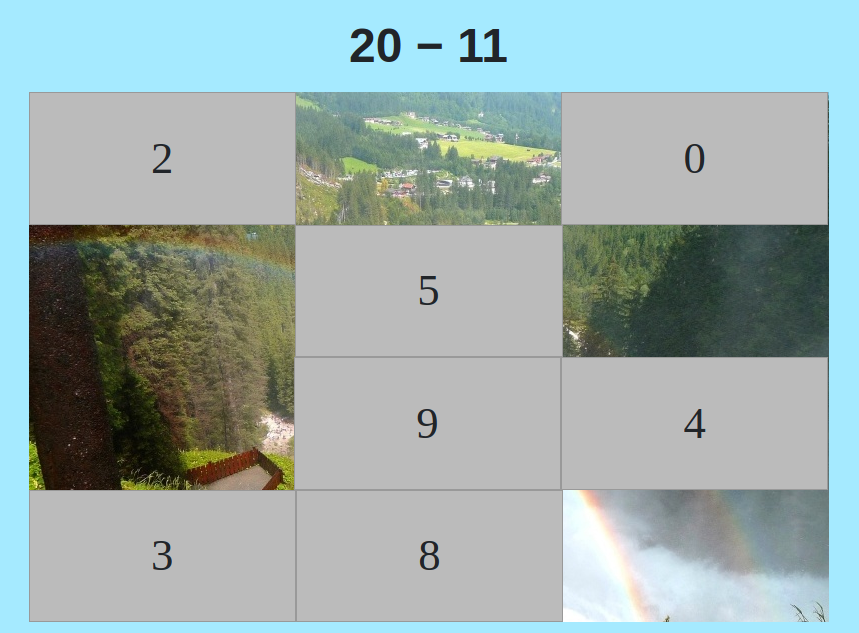MM Practice
×
Multiplication
Division
Place Value
Fractions & Decimals
Measurement
Statistics & Probability
Pre-Algebra
Money
Kindergarten
Geometry

# Subtraction Hidden Picture GameOnline practice for K/1st-4th grade

Solve simple subtraction problems while uncovering a hidden picture in this fun game that children really like!

You can choose the range of numbers to be used in the subtraction problems, which means the game can fit many grade levels. For kindergarten, choose the range 0-5. For first grade, use 0-10. For second grade, try 0-20 or 0-30. For third grade, choose 0-50, for example.

You can even use this game with middle school students to practice integer addition: simply choose a number range that involves negative numbers, such as from -10 to 10.

Screenshot:"Correct" sound credit: http://www.orangefreesounds.com